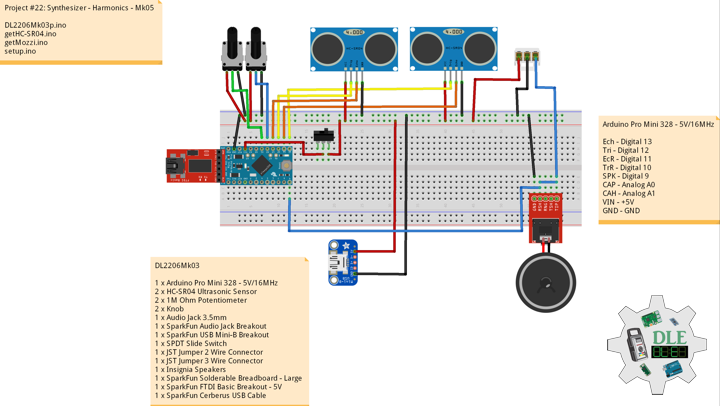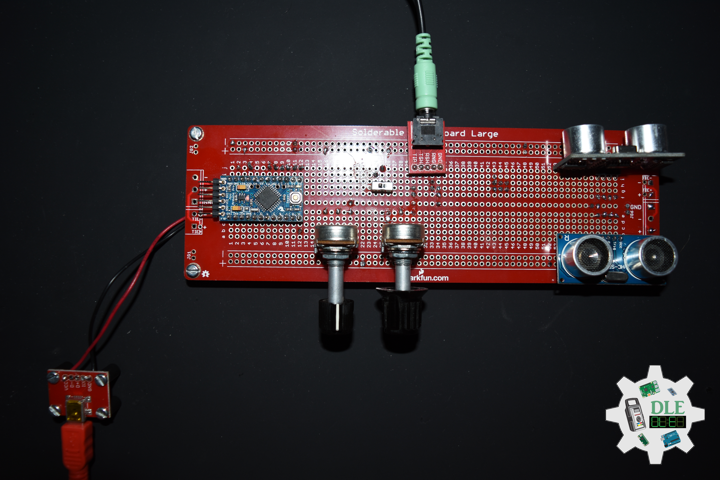The Alpha Geek – Geeking Out

# Project #22: Synthesizer – Harmonics – Mk05

——

#DonLucElectronics #DonLuc #Synthesizer #UltrasonicSynth #Mozzi #Arduino #ArduinoProMini #Project #Fritzing #Programming #Electronics #Microcontrollers #Consultant

————————

Harmonics – Brightness

Nearly all signals contain energy at harmonic frequencies, in addition to the energy at the fundamental frequency. If it contains all the energy in a signal at the fundamental frequency, then that signal is a perfect sine wave. If the signal is not a perfect sine wave, then some energy is contained in the harmonics. Some waveforms contain large amounts of energy at harmonic frequencies.

Ratio

So in the harmonic series, we have a fundamental (pitch/note) and a succession of harmonics that stem from the fundamental which also hold other pitches within themselves.

Arduino – Map

map(value, fromLow, fromHigh, toLow, toHigh)

Re-maps a number from one range to another. That is, a value of fromLow would get mapped to toLow, a value of fromHigh to toHigh, values in-between to values in-between, etc…

DL2206Mk03

1 x Arduino Pro Mini 328 – 5V/16MHz
2 x HC-SR04 Ultrasonic Sensor
2 x 1M Ohm Potentiometer
2 x Knob
1 x Audio Jack 3.5mm
1 x SparkFun Audio Jack Breakout
1 x SparkFun USB Mini-B Breakout
1 x SPDT Slide Switch
1 x JST Jumper 2 Wire Connector
1 x JST Jumper 3 Wire Connector
1 x Insignia Speakers
1 x SparkFun Solderable Breadboard – Large
1 x SparkFun FTDI Basic Breakout – 5V
1 x SparkFun Cerberus USB Cable

Arduino Pro Mini 328 – 5V/16MHz

Ech – Digital 13
Tri – Digital 12
EcR – Digital 11
TrR – Digital 10
SPK – Digital 9
CAP – Analog A0
CAH – Analog A1
VIN – +5V
GND – GND

——

DL2206Mk03p.ino

```/* ***** Don Luc Electronics © *****
Software Version Information
Project #22: Synthesizer - Harmonics - Mk05
22-05
DL2206Mk03p.ino
1 x Arduino Pro Mini 328 - 5V/16MHz
2 x HC-SR04 Ultrasonic Sensor
2 x 1M Ohm Potentiometer
2 x Knob
1 x Audio Jack 3.5mm
1 x SparkFun Audio Jack Breakout
1 x SparkFun USB Mini-B Breakout
1 x SPDT Slide Switch
1 x JST Jumper 2 Wire Connector
1 x JST Jumper 3 Wire Connector
1 x Insignia Speakers
1 x SparkFun Solderable Breadboard - Large
1 x SparkFun FTDI Basic Breakout - 5V
1 x SparkFun Cerberus USB Cable
*/

// Include the Library Code
// Mozzi
#include <MozziGuts.h>
// Oscillator
#include <Oscil.h>
// Table for Oscils to play
#include <tables/cos2048_int8.h>
// Smoothing Control
#include <Smooth.h>
// Maps unpredictable inputs to a range
#include <AutoMap.h>

// Desired carrier frequency max and min, for AutoMap
const int MIN_CARRIER_FREQ = 22;
const int MAX_CARRIER_FREQ = 440;

// Desired intensity max and min, for AutoMap, note they're inverted for reverse dynamics
const int MIN_INTENSITY = 700;
const int MAX_INTENSITY = 10;

// Desired mod speed max and min, for AutoMap, note they're inverted for reverse dynamics
const int MIN_MOD_SPEED = 10000;
const int MAX_MOD_SPEED = 1;

// Maps unpredictable inputs to a range
AutoMap kMapCarrierFreq(0,1023,MIN_CARRIER_FREQ,MAX_CARRIER_FREQ);
AutoMap kMapIntensity(0,1023,MIN_INTENSITY,MAX_INTENSITY);
AutoMap kMapModSpeed(0,1023,MIN_MOD_SPEED,MAX_MOD_SPEED);

// Set the input for the knob to analog pin 0
const int KNOB_PIN = A0;
int LDR1_PIN;
int LDR2_PIN;

// Table for Oscils to play
Oscil<COS2048_NUM_CELLS, AUDIO_RATE> aCarrier(COS2048_DATA);
Oscil<COS2048_NUM_CELLS, AUDIO_RATE> aModulator(COS2048_DATA);
Oscil<COS2048_NUM_CELLS, CONTROL_RATE> kIntensityMod(COS2048_DATA);

// Harmonics (Brightness)
int iModRatio = A1;
int mod_ratio;
// Carries control info from updateControl to updateAudio
long fm_intensity;

// Smoothing for intensity to remove clicks on transitions
float smoothness = 0.95f;
Smooth <long> aSmoothIntensity(smoothness);

// Trigger pin 12 to pitch distance sensor
const int iTrigPitch = 12;
// Echo Receive pin 13 to pitch distance sensor
const int iEchoPitch = 13;
// Define the useable range of the pitch sensor
const int pitchLowThreshold = 45;
const int pitchHighThreshold = 2;
// Stores the distance measured by the distance sensor
float distance = 0;
// Trigger pin 10 to rate distance sensor
const int iTrigRate = 10;
// Echo Receive pin 13 to pitch distance sensor
const int iEchoRate = 11;
// Define the useable range of the pitch sensor
const int rateLowThreshold = 45;
const int rateHighThreshold = 2;
// Stores the distance measured by the distance sensor
float rate = 0;

// Mini Speaker
int SPK = 9;

// Software Version Information
String sver = "22-05";

void loop() {

// Audio Hook
audioHook();

}
```

getHC-SR04.ino

```// HC-SR04 Ultrasonic Sensor
// Setup HC-SR04
void setupHCSR04() {

// The trigger iTrig Pitch will output pulses of electricity
pinMode(iTrigPitch, OUTPUT);
// The echo iEcho will measure the duration of pulses coming back from the distance sensor
pinMode(iEchoPitch, INPUT);

// The trigger iTrig Rate will output pulses of electricity
pinMode(iTrigRate, OUTPUT);
// The echo iEcho will measure the duration of pulses coming back from the distance sensor
pinMode(iEchoRate, INPUT);

}
// Distance
float isDistance() {

// Variable to store the time it takes for a ping to bounce off an object
float echoTime;
// Variable to store the distance calculated from the echo time
float calculatedDistance;

// Send out an ultrasonic pulse that's 10ms long
digitalWrite(iTrigPitch, HIGH);
delayMicroseconds(10);
digitalWrite(iTrigPitch, LOW);

// Use the pulseIn command to see how long it takes for the
// pulse to bounce back to the sensor
echoTime = pulseIn(iEchoPitch, HIGH);

// Calculate the distance of the object that reflected the pulse
// (half the bounce time multiplied by the speed of sound)
calculatedDistance = echoTime * 0.034 / 2;

// Send back the distance that was calculated
return calculatedDistance;

}
// Rate
float isRate() {

// Variable to store the time it takes for a ping to bounce off an object
float echoTime;
// Variable to store the distance calculated from the echo time
float calculatedDistance;

// Send out an ultrasonic pulse that's 10ms long
digitalWrite(iTrigRate, HIGH);
delayMicroseconds(10);
digitalWrite(iTrigRate, LOW);

// Use the pulseIn command to see how long it takes for the
// pulse to bounce back to the sensor
echoTime = pulseIn(iEchoRate, HIGH);

// Calculate the distance of the object that reflected the pulse
// (half the bounce time multiplied by the speed of sound)
// cm = 58.0
calculatedDistance = echoTime * 0.034 / 2;

// Send back the distance that was calculated
return calculatedDistance;

}
```

getMozzi.ino

```// Mozzi
// Update Control
void updateControl(){

// Variable to store the distance measured by the sensor
distance = isDistance();
// Low Threshold
if ( distance >= pitchLowThreshold) {

// pitchLowThreshold
distance = pitchLowThreshold;

}
// High Threshold
if ( distance < pitchHighThreshold){

// pitchHighThreshold
distance = pitchHighThreshold;

}

// Variable to store the distance measured by the sensor
rate = isRate();
// Low Threshold
if ( rate >= rateLowThreshold) {

// rateLowThreshold
rate = rateLowThreshold;

}
// High Threshold
if ( rate < rateHighThreshold){

// rateHighThreshold
rate = rateHighThreshold;

}

// Map
distance = map(distance, 45, 2, 0, 1023);
rate = map(rate, 45, 2, 0, 1023);

// Value is 0-1023

// Value is 0-1023

// Map
mod_ratio = map(mod_ratio, 0, 1023, 2, 15);

// Map the knob to carrier frequency
int carrier_freq = kMapCarrierFreq(knob_value);

// Calculate the modulation frequency to stay in ratio
int mod_freq = carrier_freq * mod_ratio;

// Set the FM oscillator frequencies
aCarrier.setFreq(carrier_freq);
aModulator.setFreq(mod_freq);

// Read the light dependent resistor on the width
LDR1_PIN = distance;
int LDR1_value = LDR1_PIN;

int LDR1_calibrated = kMapIntensity(LDR1_value);

// Calculate the fm_intensity
// Shift back to range after 8 bit multiply
fm_intensity = ((long)LDR1_calibrated * (kIntensityMod.next()+128))>>8;

// Read the light dependent resistor on the speed
LDR2_PIN = rate;
int LDR2_value= LDR2_PIN;

// Use a float here for low frequencies
float mod_speed = (float)kMapModSpeed(LDR2_value)/1000;

kIntensityMod.setFreq(mod_speed);

}
// Update Audio
int updateAudio()
{

// Update Audio
long modulation = aSmoothIntensity.next(fm_intensity) * aModulator.next();
return aCarrier.phMod(modulation);

}
```

setup.ino

```// Setup
void setup() {

// Setup HC-SR04
setupHCSR04();

// Delay
delay( 200 );

// Mozzi Start
startMozzi();

}
```

——

Technology Experience

• Single-Board Microcontrollers (PIC, Arduino, Raspberry Pi,Espressif, etc…)
• IoT
• Robotics
• Camera and Video Capture Receiver Stationary, Wheel/Tank and Underwater Vehicle
• Unmanned Vehicles Terrestrial and Marine
• Research & Development (R & D)

Instructor and E-Mentor

• IoT
• PIC Microcontrollers
• Arduino
• Raspberry Pi
• Espressif
• Robotics